# 傻子都能看懂的floyd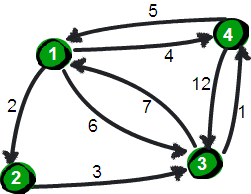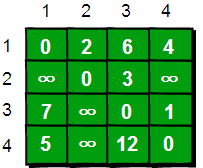for (i = 1; i <= n; i++) {
for (j = 1; j <= n; j++) {
if (e[i][j] > e[i] + e[j])
e[i][j] = e[i] + e[j];
}
}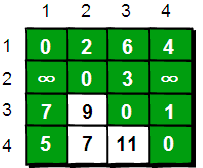//经过1号顶点
for(i=1;i<=n;i++)
for(j=1;j<=n;j++)
if (e[i][j] > e[i]+e[j])
e[i][j]=e[i]+e[j];
//经过2号顶点
for(i=1;i<=n;i++)
for(j=1;j<=n;j++)
if (e[i][j] > e[i]+e[j])
e[i][j]=e[i]+e[j];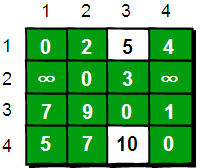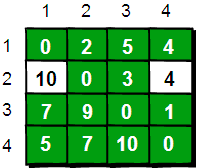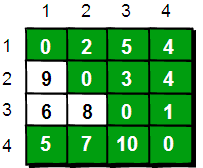for(k=1;k<=n;k++)
for(i=1;i<=n;i++)
for(j=1;j<=n;j++)
if(e[i][j]>e[i][k]+e[k][j])
e[i][j]=e[i][k]+e[k][j];



int main()  {
int e,k,i,j,n,m,t1,t2,t3;
int inf=99999999; //用inf(infinity的缩写)存储一个我们认为的正无穷值
//读入n和m，n表示顶点个数，m表示边的条数
scanf("%d %d",&n,&m);
//初始化
for(i=1;i<=n;i++)
for(j=1;j<=n;j++)
if(i==j) e[i][j]=0;
else e[i][j]=inf;
//读入边
for(i=1;i<=m;i++) {
scanf("%d %d %d",&t1,&t2,&t3);
e[t1][t2]=t3;
}
//Floyd-Warshall算法核心语句
for(k=1;k<=n;k++)
for(i=1;i<=n;i++)
for(j=1;j<=n;j++)
if(e[i][j]>e[i][k]+e[k][j] )
e[i][j]=e[i][k]+e[k][j];
//输出最终的结果
for(i=1;i<=n;i++){
for(j=1;j<=n;j++)
printf("%10d",e[i][j]);
printf("\n");
}
return 0;
}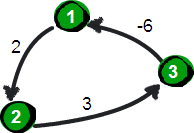posted @ 2020-04-29 16:30  _Destiny  阅读(...)  评论(...编辑  收藏